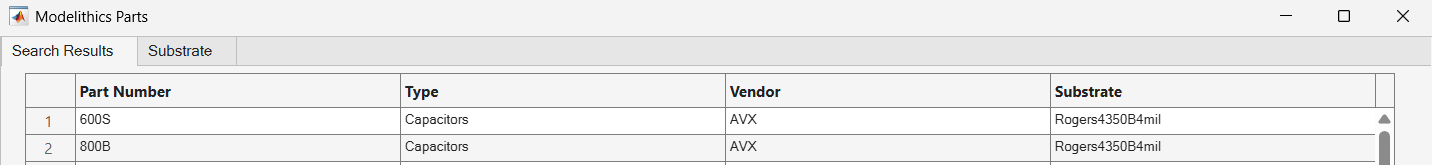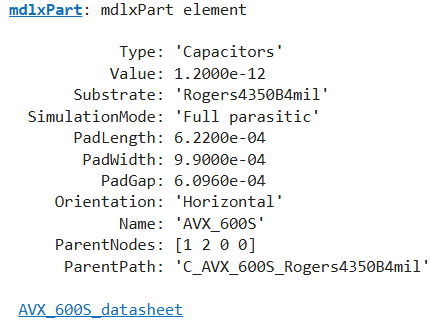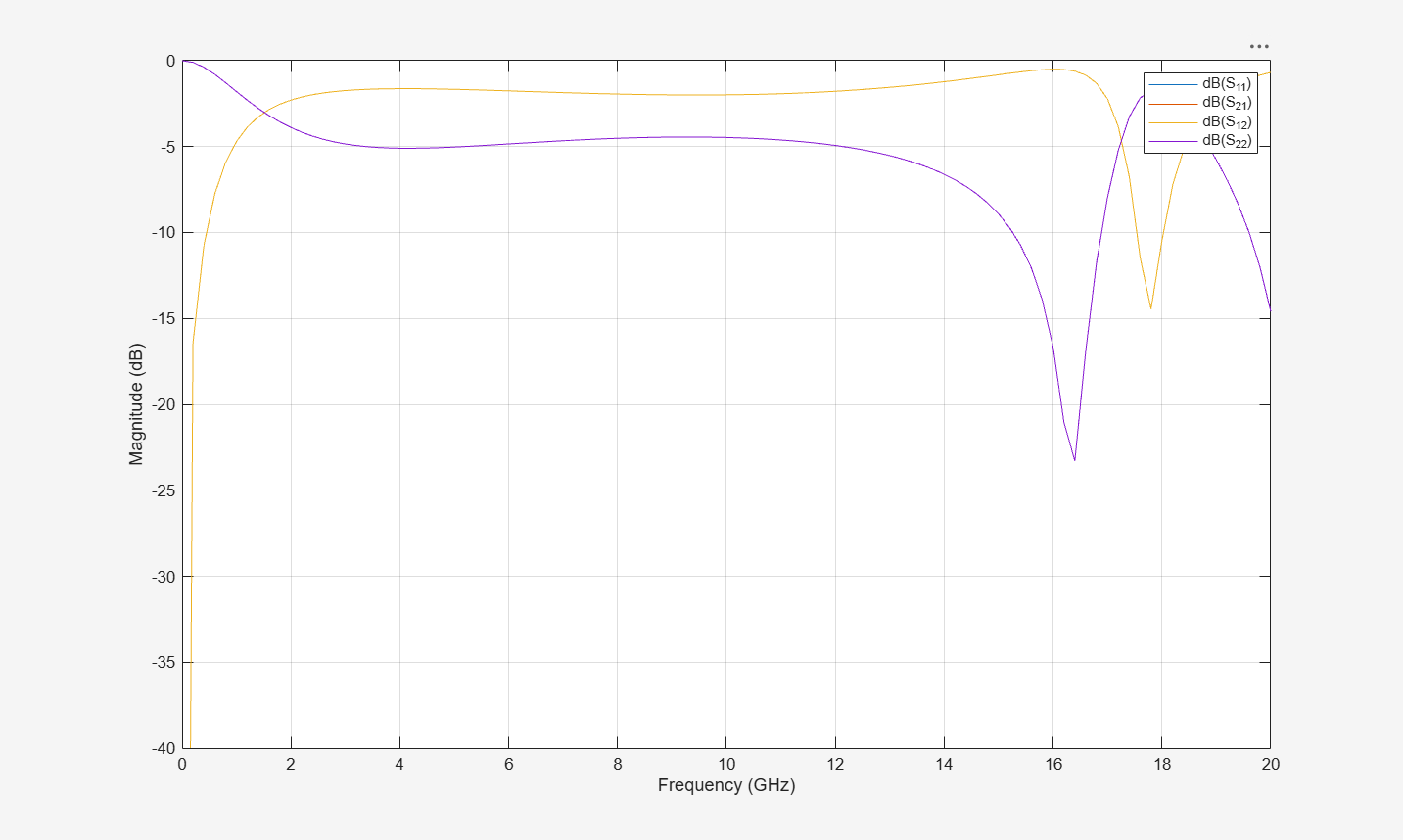# mdlxSetup

Set up Modelithics library

Since R2023a

## Syntax

`` mdlxSetup(libpath)``

## Description

example

```` mdlxSetup(libpath)` sets up the Modelithics® library specified in `libpath`. `libpath` is persistent across MATLAB® sessions. NoteThis function requires a Modelithics SELECT+ Library™ license. For more information, see Modelithics SELECT+ Library for MATLAB. ```

## Examples

collapse all

Set up Modelithics SELECT+ Library™.

```mdlxSetup('C:\mdlx_library\SELECT') ```

Create a new Modelithics library object related to the library you set up.

```mdlx = mdlxLibrary; ```

Search the library for a component that you can mount on the `Rogers4350B4mil` substrate with the vendor name `ATC`.

```search(mdlx,'Rogers4350B4mil',Vendor='ATC') ```The ATC 600S capacitor on a 4 mil Roger 4350B substrate fits the search criteria. Create a Modelithics component object that matches these characteristics.

```pobj = mdlxPart(mdlx,'600S','Capacitors','ATC','Rogers4350B4mil',Value=1.2e-12); ```

Create a circuit named `C_ATC_600S_Rogers4350B4mil`.

```hckt = circuit('C_ATC_600S_Rogers4350B4mil') ```Add the Modelithics component to the circuit.

```add(hckt,[1 2],pobj); ```

Set the ports of the circuit.

```setports(hckt, [1 0],[2 0]); ```

Display the Modelithics component.

```disp(pobj) ```Calculate the S-parameters of the circuit.

```S = sparameters(hckt,freq); ```

Plot the S-parameters characteristics.

```figure; rfplot(S) ```## Input Arguments

collapse all

Modelithics library folder path, specified as a string scalar.

## Version History

Introduced in R2023a